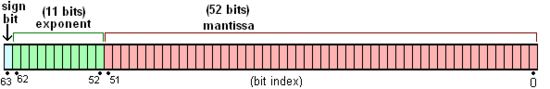# js中小数四舍五入和浮点数的研究

## 浮点数运算后的精度问题

```// 加法 =====================
0.1 + 0.2 = 0.30000000000000004
0.7 + 0.1 = 0.7999999999999999
0.2 + 0.4 = 0.6000000000000001

// 减法 =====================
1.5 - 1.2 = 0.30000000000000004
0.3 - 0.2 = 0.09999999999999998

// 乘法 =====================
19.9 * 100 = 1989.9999999999998
0.8 * 3 = 2.4000000000000004
35.41 * 100 = 3540.9999999999995

// 除法 =====================
0.3 / 0.1 = 2.9999999999999996
0.69 / 10 = 0.06899999999999999
```

## toFixed奇葩问题

```1.35.toFixed(1) // 1.4 正确
1.335.toFixed(2) // 1.33 错误
1.3335.toFixed(3) // 1.333 错误
1.33335.toFixed(4) // 1.3334 正确
1.333335.toFixed(5)  // 1.33333 错误
1.3333335.toFixed(6) // 1.333333 错误
```

## 浮点数的存储

• 符号位S：第 1 位是正负数符号位（sign），0代表正数，1代表负数

• 指数位E：中间的 11 位存储指数（exponent），用来表示次方数

• 尾数位M：最后的 52 位是尾数（mantissa），超出的部分自动进一舍零## 浮点数的运算

```0.1 -> 0.0001 1001 1001 1001...(1100循环)
0.2 -> 0.0011 0011 0011 0011...(0011循环)
```
IEEE 754 标准的 64 位双精度浮点数的小数部分最多支持53位二进制位，所以两者相加之后得到二进制为：
```0.0100110011001100110011001100110011001100110011001100
```

## 解决toFixed

```// toFixed兼容方法
Number.prototype.toFixed = function(len){
if(len>20 || len<0){
throw new RangeError('toFixed() digits argument must be between 0 and 20');
}
// .123转为0.123
var number = Number(this);
if (isNaN(number) || number >= Math.pow(10, 21)) {
return number.toString();
}
if (typeof (len) == 'undefined' || len == 0) {
return (Math.round(number)).toString();
}
var result = number.toString(),
numberArr = result.split('.');

if(numberArr.length<2){
//整数的情况
}
var intNum = numberArr, //整数部分
deciNum = numberArr,//小数部分
lastNum = deciNum.substr(len, 1);//最后一个数字

if(deciNum.length == len){
//需要截取的长度等于当前长度
return result;
}
if(deciNum.length < len){
//需要截取的长度大于当前长度 1.3.toFixed(2)
}
//需要截取的长度小于当前长度，需要判断最后一位数字
result = intNum + '.' + deciNum.substr(0, len);
if(parseInt(lastNum, 10)>=5){
//最后一位数字大于5，要进位
var times = Math.pow(10, len); //需要放大的倍数
var changedInt = Number(result.replace('.',''));//截取后转为整数
changedInt++;//整数进位
changedInt /= times;//整数转为小数，注：有可能还是整数
}
return result;
//对数字末尾加0
var dotPos = num.indexOf('.');
if(dotPos === -1){
//整数的情况
num += '.';
for(var i = 0;i<len;i++){
num += '0';
}
return num;
} else {
//小数的情况
var need = len - (num.length - dotPos - 1);
for(var j = 0;j<need;j++){
num += '0';
}
return num;
}
}
}
```

## 解决浮点数运算精度

```0.1 + 0.2 == 0.3 //false
(0.1*10 + 0.2*10)/10 == 0.3 //true
```

```35.41 * 100 = 3540.9999999999995
```

``` /*** method **
*  add / subtract / multiply /divide
* floatObj.multiply(19.9, 100) >> 1990
*
*/
var floatObj = function(){

/*
* 判断obj是否为一个整数
*/
function isInteger(obj){
return Math.floor(obj) === obj
}

/*
* 将一个浮点数转成整数，返回整数和倍数。如 3.14 >> 314，倍数是 100
* @param floatNum {number} 小数
* @return {object}
*   {times:100, num: 314}
*/
function toInteger(floatNum){
var ret = {times: 1, num: 0}
if (isInteger(floatNum)) {
ret.num = floatNum
return ret
}
var strfi  = floatNum + ''
var dotPos = strfi.indexOf('.')
var len    = strfi.substr(dotPos+1).length
var times  = Math.pow(10, len)
var intNum = Number(floatNum.toString().replace('.',''))
ret.times  = times
ret.num    = intNum
return ret
}

/*
* 核心方法，实现加减乘除运算，确保不丢失精度
* 思路：把小数放大为整数（乘），进行算术运算，再缩小为小数（除）
*
* @param a {number} 运算数1
* @param b {number} 运算数2
* @param digits {number} 精度，保留的小数点数，比如 2, 即保留为两位小数
*
*/
function operation(a, b, digits, op){
var o1 = toInteger(a)
var o2 = toInteger(b)
var n1 = o1.num
var n2 = o2.num
var t1 = o1.times
var t2 = o2.times
var max = t1 > t2 ? t1 : t2
var result = null
switch (op) {
if (t1 === t2) { // 两个小数位数相同
result = n1 + n2
} else if (t1 > t2) { // o1 小数位 大于 o2
result = n1 + n2 * (t1 / t2)
} else { // o1 小数位 小于 o2
result = n1 * (t2 / t1) + n2
}
return result / max
case 'subtract':
if (t1 === t2) {
result = n1 - n2
} else if (t1 > t2) {
result = n1 - n2 * (t1 / t2)
} else {
result = n1 * (t2 / t1) - n2
}
return result / max
case 'multiply':
result = (n1 * n2) / (t1 * t2)
return result
case 'divide':
result = (n1 / n2) * (t2 / t1)
return result
}
}

// 加减乘除的四个接口
}
function subtract(a, b, digits){
return operation(a, b, digits, 'subtract')
}
function multiply(a, b, digits){
return operation(a, b, digits, 'multiply')
}
function divide(a, b, digits){
return operation(a, b, digits, 'divide')
}

// exports
return {